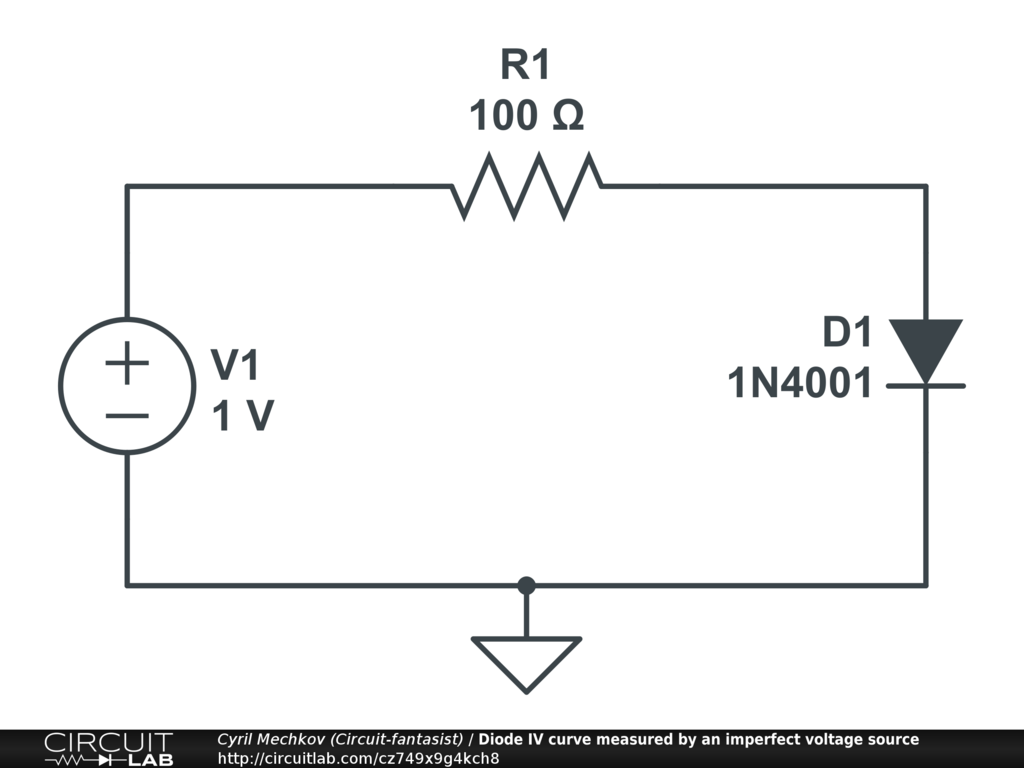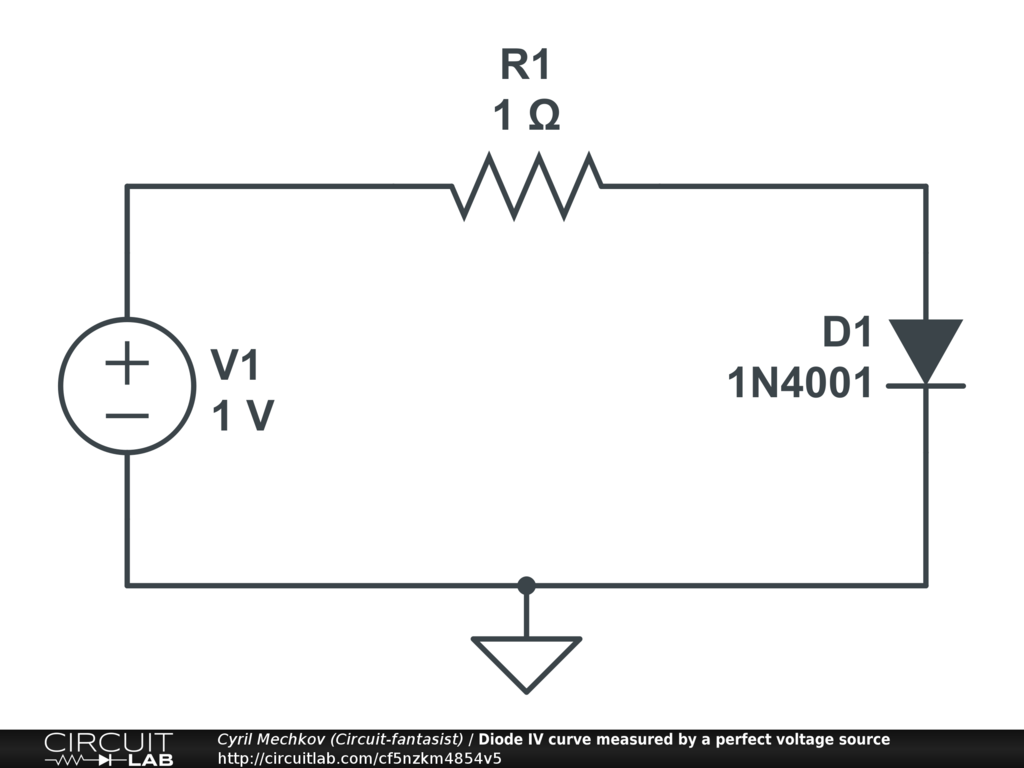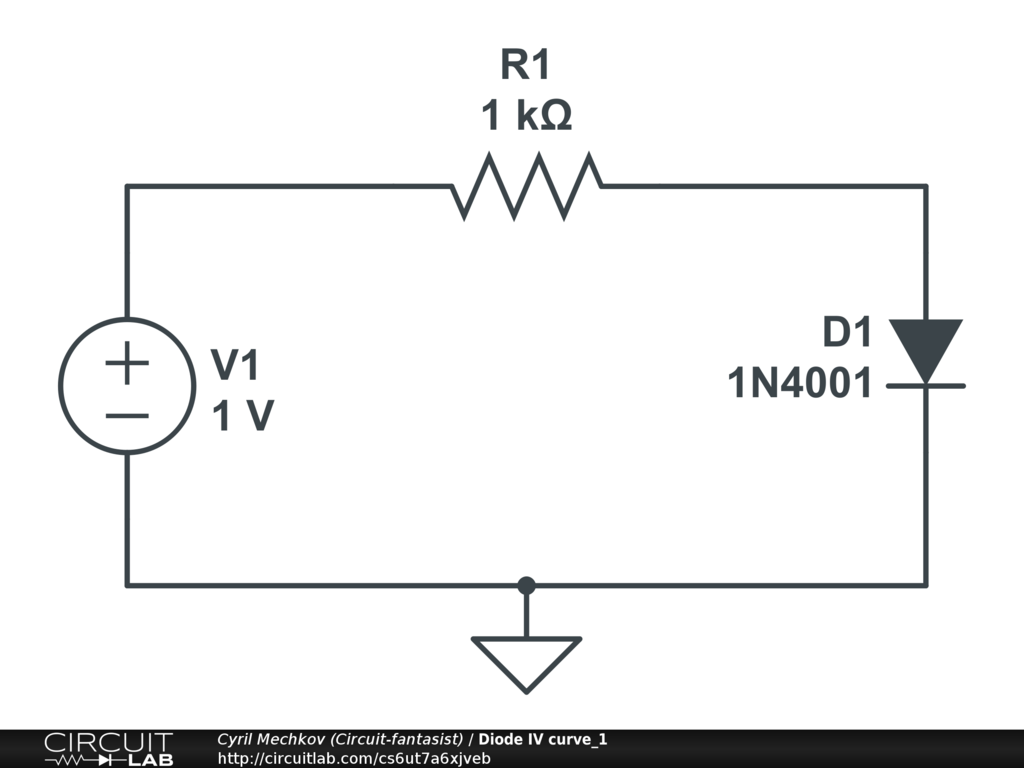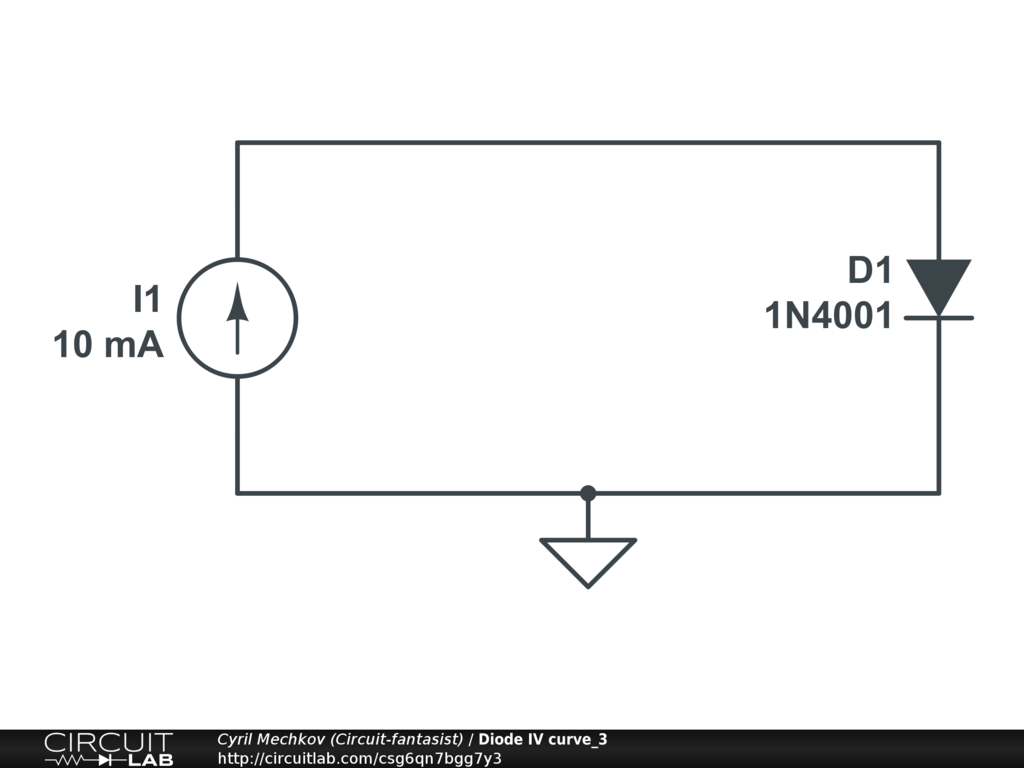## How do we plot diode IV curves by the DC Sweep simulation?

 For years, I used a simple but accurate curve tracer in labs on Semiconductor Devices with my students (I have described it in a story in my blog Circuit Stories). So it was interesting to me to get acquainted with the DC Sweep mode of CircuitLab described in Lecture 3 of CircuitLab Academy. There are some subtle moments in conducting this experiment that I want to share with you. The role of the resistor R1. In the vertical part of its curve, the diode behaves as a voltage stabilizer. We can imagine it as a "dynamic resistor" that changes its resistance in the opposite direction to current changes so V = R.I = const. When we connect a varying voltage source to the diode, a conflict may occur between the two "voltage devices" and the current may increase unacceptably. So the role of the resistor is to limit the current (to make the ideal voltage source real). The problem is, however, that the voltage drop across it can introduce an error when added to the forward diode voltage drop. This is the case here where the entire input voltage and not just the diode voltage is used as the independent variable on the abscissa. Thus we actually get the much flatter IV curve of the composite element D1 + R1… and not only of D1. And when we replace the real diode with an ideal one (at the end of the lecture) we actually get the IV curve of the resistor and not of the diode… which is quite confusing. How can we avoid the error introduced by the resistor?In the curve tracer above, this problem is elegantly solved by making the input voltage source floating and the midpoint between the diode and resistor grounded. So the true voltage drops across the diode and resistor are applied to the XY scope inputs. In CircuitLab, this means that the voltage across the diode (not the input) should be applied along the abscissa... but it seems that there is no such possibility. Then we can do the following: Eliminating the resistor R1 (ideal voltage source). We can reduce its resistance to zero (or 1 ohm) and carefully begin to increase the input voltage, making sure that the current does not exceed the maximum allowable. This is how we determine the maximum voltage of the DC sweep and set it in the parameters. In this way, we will explore the diode by an "ideal" voltage source without any error.Increasing both voltage and resistance (real current source). As an output value we now set the voltage across the diode. Unfortunately, the curve turns out to be rotated and mirrored.Sweeping by an ideal current source. This is considered the best way to study diode elements. But here, too, the curve will turn out to be inverted and mirrored.In the empty places I have inserted images of the graphs enclosed with tags but I don't know why they are not visible). by Circuit-fantasist February 14, 2023